DataWorks allows you to configure cross-cycle scheduling dependencies for auto triggered nodes. You can configure the instance generated for a node in the current cycle to depend on the instances generated for one or more specific nodes in the previous cycle. This way, the instance generated for the node in the current cycle can start to run only after the instances generated for one or more specific nodes on which the node depends are successfully run. This topic describes how to configure cross-cycle scheduling dependencies for a node and the types of cross-cycle dependencies.

Configure scheduling dependencies

Create a node in DataStudio and go to the configuration tab of the node. Click the Properties tab in the right-side navigation pane. Then, configure scheduling dependencies for the node in the Dependencies section of the Properties tab. DataWorks allows you to configure same-cycle or cross-cycle scheduling dependencies for a node.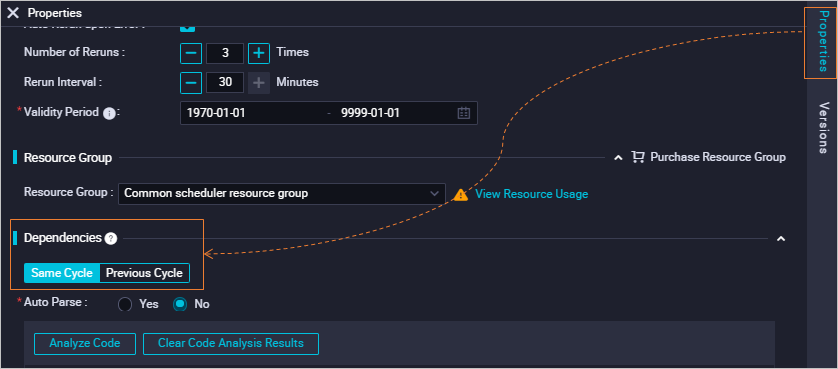The following table describes the differences between the two types of scheduling dependencies.
Dependency type Business logic and use scenario Display of dependencies
Same-cycle scheduling dependency The current node (Node A) needs to use the table data that is generated by another node on the current day. The table is specified in the SELECT statement for Node A. To ensure that Node A can successfully obtain the table data, you must configure same-cycle scheduling dependencies for Node A. Same-cycle scheduling dependencies are presented as solid lines in Operation Center. For more information about how to go to Operation Center and view the scheduling dependencies of a node, see Overview.
Cross-cycle scheduling dependency The current node (Node A) needs to use the table data that is generated by one or more specific nodes in the previous cycle. The table is specified in the SELECT statement for Node A. To ensure that Node A can successfully obtain the table data, you must configure cross-cycle scheduling dependencies for Node A. Cross-cycle scheduling dependencies are presented as dashed lines in Operation Center. For more information about how to go to Operation Center and view the scheduling dependencies of a node, see Overview.

Types of cross-cycle scheduling dependencies

The following table describes the types of cross-cycle scheduling dependencies.
Type Description Scenario
Dependency on the instance generated for the current node in the previous cycle

The instance generated for a node in the current cycle starts to run only after the instance generated for the same node in the previous cycle is successfully run.

The instance generated for a node in the current cycle depends on the latest business data from the instance generated for the same node in the previous cycle.

Dependency on the instances generated for the descendant nodes of a node in the previous cycle

The instance generated for a node in the current cycle starts to run only after the instances generated for the descendant nodes of the current node in the previous cycle are successfully run.

For example, Node A has three descendant nodes: Node B, Node C, and Node D. If you configure this type of scheduling dependency for Node A, the instance generated for Node A in the current cycle depends on the instances generated for Node B, Node C, and Node D in the previous cycle. The instance generated for Node A in the current cycle starts to run only after the instances generated for Node B, Node C, and Node D in the previous cycle are successfully run.

The instance generated for a node in the current cycle depends on whether the output table data of the current node in the previous cycle is cleansed by the instances generated for the descendant nodes of the current node in the previous cycle.

Dependency on the instances generated for one or more specified nodes in the previous cycle
The instance generated for a node in the current cycle starts to run only after the instances generated for one or more specified nodes in the previous cycle are successfully run.
Note If you configure this type of scheduling dependency for a node, you must search by node ID or node name to add the nodes on which the node needs to depend in the Dependencies section of the Properties tab.

The instance generated for a node in the current cycle depends on the output table data from the instances generated for one or more other nodes in the previous cycle in the business logic but does not use the data in the code.

You can set Follow the upstream air running attribute to Yes when you set Depend On to Instances of Current Node or Other Nodes. For more information, see Pass the dry-run attribute of an ancestor node.

Dependency on the instance generated for the current node in the previous cycle

• Node dependency

The instance generated for a node in the current cycle starts to run only after the instance generated for the same node in the previous cycle is successfully run.

• Scenario

The instance generated for a node in the current cycle depends on the latest business data from the instance generated for the same node in the previous cycle.

• Impact on the scheduling of the current node whose instance generated in the current cycle is configured to depend on the instance generated for the same node in the previous cycle
• Example 1: Configure the instance generated in the current cycle for a node scheduled by day to depend on the instance generated for the same node in the previous cycle
• WorkflowRoot and Node_A are auto triggered nodes that are scheduled by day.
• The following configuration is performed for Node_A: The instance generated in the current cycle depends on the instance generated in the previous cycle.
• Node_A generates an instance named Instance_A in the current cycle (T).
• Node_A generates an instance named Instance_A' in the previous cycle (T-1).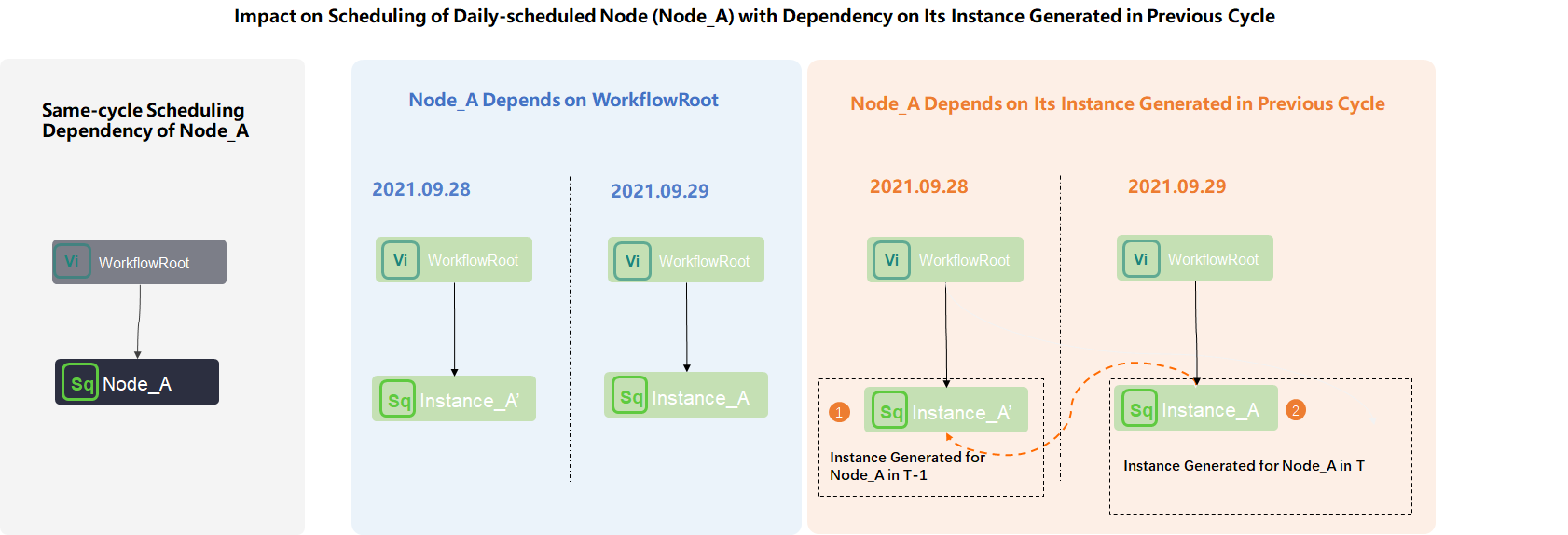After you set Depend On to Instances of Current Node for Node_A in the Dependencies section of the Properties tab, Instance_A starts to run in the current cycle only after Instance_A' and the instance generated for WorkflowRoot in the current cycle are successfully run.
• Example 2: Configure the instance generated in the current cycle for a node scheduled by hour to depend on the instance generated for the same node in the previous cycle
• WorkflowRoot is a zero load node that is scheduled by day. Node_A is an auto triggered node that is scheduled by hour. WorkflowRoot is the ancestor node of the Node_A.
• Node_A is scheduled to run every 8 hours from 00:00 to 23:59. The scheduled time of Node_A is 00:00 (T1), 08:00 (T2), and 16:00 (T3).
• Node_A generates the following instances at T1, T2, and T3: Instance_A1, Instance_A2, and Instance_A3.
• The following configuration is performed for Node_A: The instance generated in the current cycle depends on the instance generated in the previous cycle.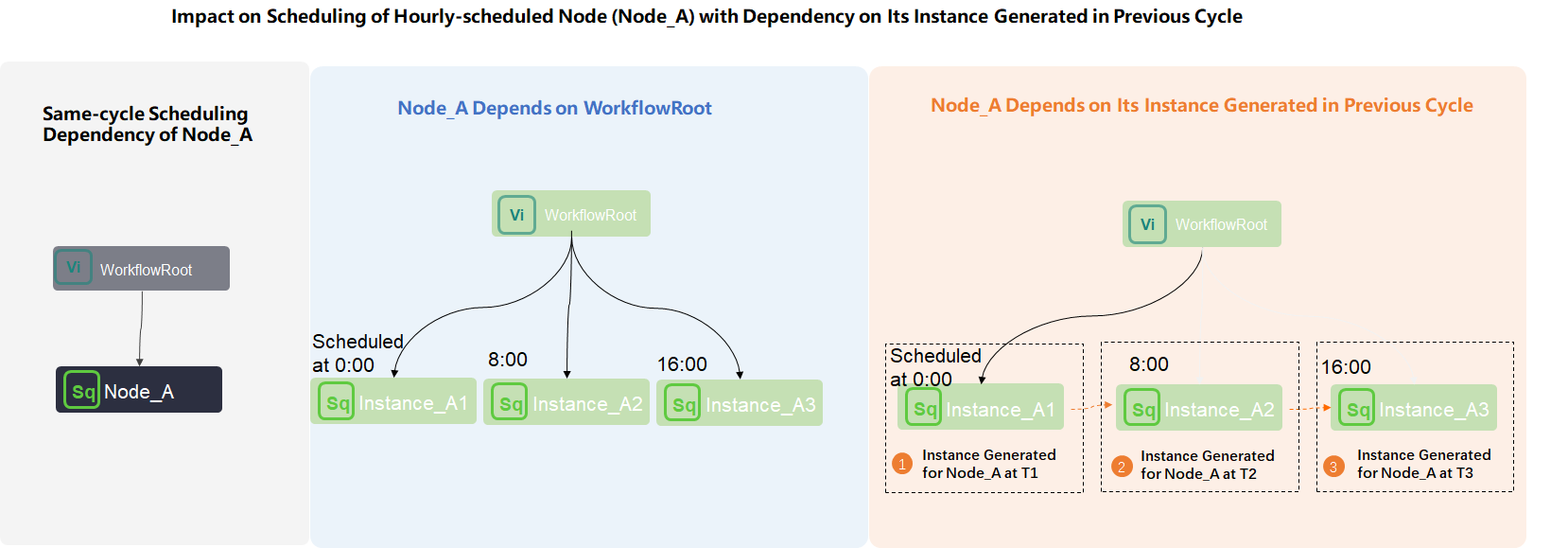• If you do not configure the instance generated for Node_A in the current cycle to depend on the instance generated for Node_A in the previous cycle, after WorkflowRoot is successfully run on the current day, Instance_A1, Instance_A2, and Instance_A3 run based on their own scheduled time.
• If configure the instance generated for Node_A in the current cycle to depend on the instance generated for Node_A in the previous cycle, Instance_A1 depends on the instance generated for WorkflowRoot, Instance_A2 depends on Instance_A1, and Instance_A3 depends on Instance_A2. In this case, an instance can run only after the instance on which it depends in the previous cycle is successfully run.
Note This example uses an auto triggered node scheduled by hour to demonstrate the logic of the dependency on the instance generated for the same node in the previous cycle. The logic is similar for an auto triggered node scheduled by minute.
• Impact on the scheduling of the descendant nodes when the instance generated in the current cycle for the current node scheduled by hour or minute to depend on the instance generated for the same node in the previous cycle
• WorkflowRoot is a zero load node scheduled by day. Node_A is an auto triggered node scheduled by hour. WorkflowRoot is the ancestor node of Node_A. Node_B and Node_C are auto triggered nodes scheduled by day and are descendant nodes of Node_A.
• Node_A is scheduled to run every 8 hours from 00:00 to 23:59. The scheduled time of Node_A is 00:00 (T1), 08:00 (T2), and 16:00 (T3).
• Node_B is scheduled to run at 00:00 every day. Node_C is scheduled to run at 08:00 every day.
• Node_A generates the following instances at T1, T2, and T3: Instance_A1, Instance_A2, and Instance_A3.
• Node_B and Node_C generate instances Instance_B and Instance_C.
• The following configuration is performed for Node_A: The instance generated in the current cycle depends on the instance generated in the previous cycle.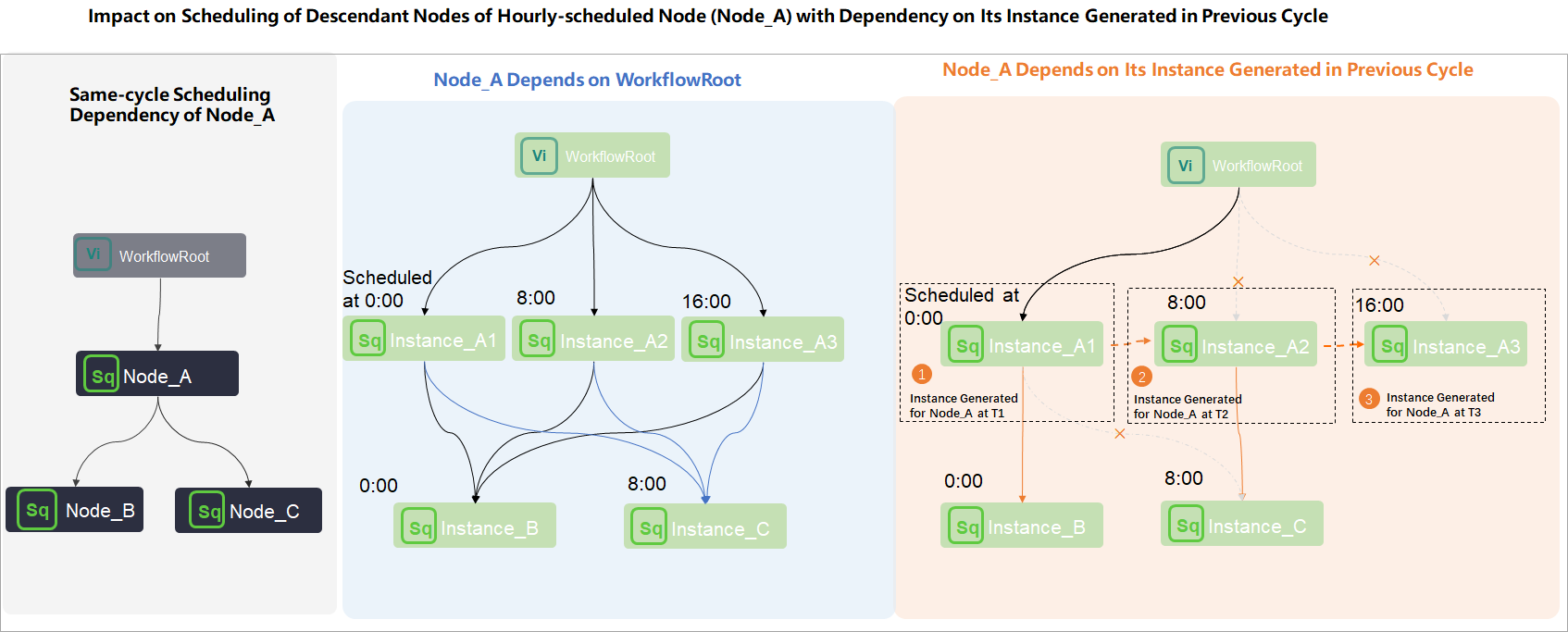• If you do not configure the instance generated for Node_A in the current cycle to depend on the instance generated for Node_A in the previous cycle, the instances generated for Node_A, Node_B, and Node_C run in the following ways:
• After WorkflowRoot is successfully run on the current day, Instance_A1, Instance_A2, and Instance_A3 run based on their own scheduled time.
• Instance_B and Instance_C depend on all the three instances generated for Node_A on the current day. This indicates that Instance_B and Instance_C start to run only after Instance_A1, Instance_A2, and Instance_A3 are all successfully run on the current day.
• If you configure the instance generated for Node_A in the current cycle to depend on the instance generated for Node_A in the previous cycle, the instances generated for Node_A, Node_B, and Node_C run in the following ways:
• Instance_A1 depends on the instance generated for WorkflowRoot, Instance_A2 depends on Instance_T1, and Instance_A3 depends on Instance_A2. In this case, an instance can run only after the instance on which it depends in the previous cycle is successfully run.
• Instance_B and Instance_C depend on the instances whose scheduled time is closest to their scheduled time.

This indicates that Instance_B whose scheduled time is 00:00 starts to run after Instance_A1 is successfully run. Instance_C does not run.

Instance_C whose scheduled time is 08:00 starts to run after Instance_A2 is successfully run.

Dependency on the instances generated for the descendant nodes of a node in the previous cycle

• Node dependency

The instance generated for a node in the current cycle starts to run only after the instances generated for the descendant nodes of the current node in the previous cycle are successfully run.

For example, Node A has three descendant nodes: Node B, Node C, and Node D. If you configure this type of scheduling dependency for Node A, the instance generated for Node A in the current cycle depends on the instances generated for Node B, Node C, and Node D in the previous cycle. The instance generated for Node A in the current cycle starts to run only after the instances generated for Node B, Node C, and Node D in the previous cycle are successfully run.

• Scenario

The instance generated for a node in the current cycle depends on whether the output table data of the current node in the previous cycle is cleansed by the instances generated for the descendant nodes of the current node in the previous cycle.

• Example
• WorkflowRoot, Node_A, Node_B, and Node_C are auto triggered nodes scheduled by day.
• Node_B and Node_C are the descendant nodes of Node_A.
• Node_A, Node_B, and Node_C generate the following instances in the current cycle (T): Instance_A, Instance_B, and Instance_C.
• Node_A, Node_B, and Node_C generate the following instances in the previous cycle (T-1): Instance_A', Instance_B', and Instance_C'.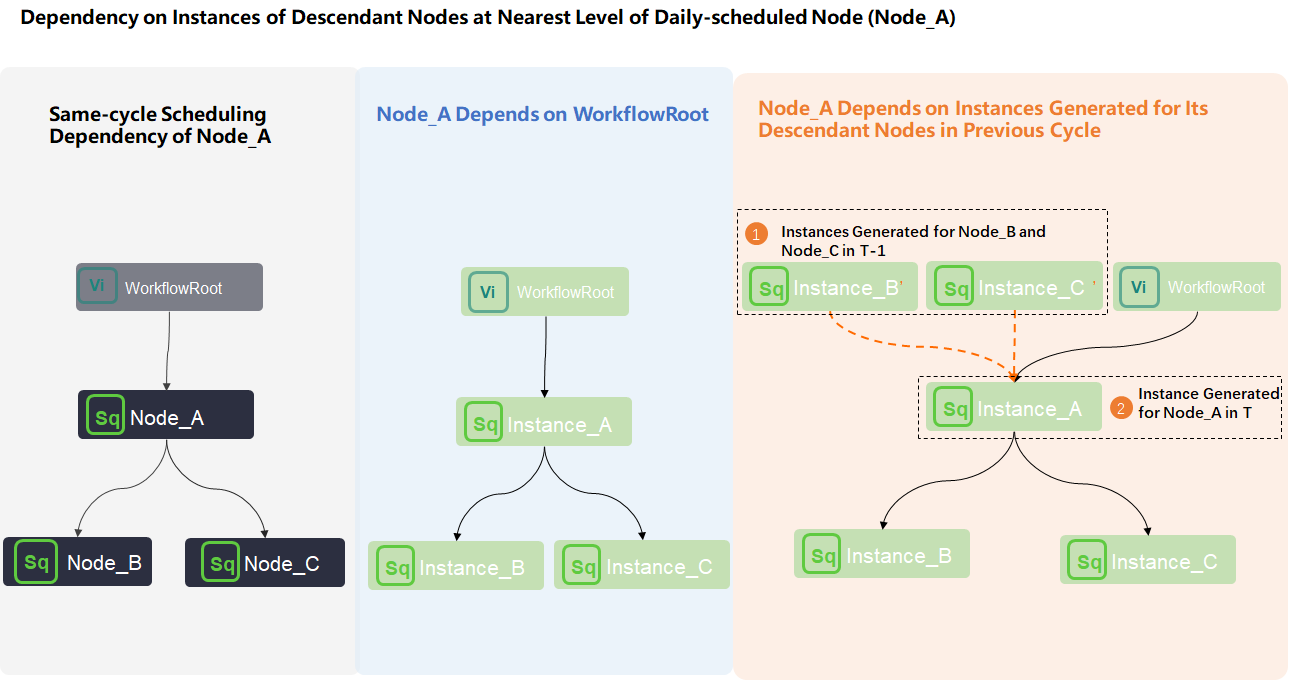After you set Depend On to Level-1 Child Node for Node_A, Instance_A depends on Instance_B', Instance_C', and the instance generated for WorkflowRoot. In this case, Instance_A starts to run only after Instance_B', Instance_C', and the instance generated for WorkflowRoot are successfully run.

Dependency on the instances generated for one or more specified nodes in the previous cycle

• Node dependency
The instance generated for a node in the current cycle starts to run only after the instances generated for one or more specified nodes in the previous cycle are successfully run.
Note If you configure this type of scheduling dependency for a node, you must search by node ID or node name to add the nodes on which the node needs to depend in the Dependencies section of the Properties tab.
• Scenario

The instance generated for a node in the current cycle depends on the output table data from the instances generated for one or more other nodes in the previous cycle in the business logic but does not use the data in the code.

• Example
• WorkflowRoot_1, WorkflowRoot_2, Node_A, Node_B, and Node_C are auto triggered nodes scheduled by day.
• Node_A, Node_B, and Node_C belong to different workflows.

Node_A and Node_B are the descendant nodes of WorkflowRoot_1. Node_C is the descendant node of WorkflowRoot_2.

• The following configuration is performed for Node_A: The instance generated for Node_A in the current cycle depends on the instance generated for Node_C in the previous cycle.
• Node_A, Node_B, and Node_C generate the following instances in the current cycle (T): Instance_A, Instance_B, and Instance_C.
• Node_A, Node_B, and Node_C generate the following instances in the previous cycle (T-1): Instance_A', Instance_B', and Instance_C'.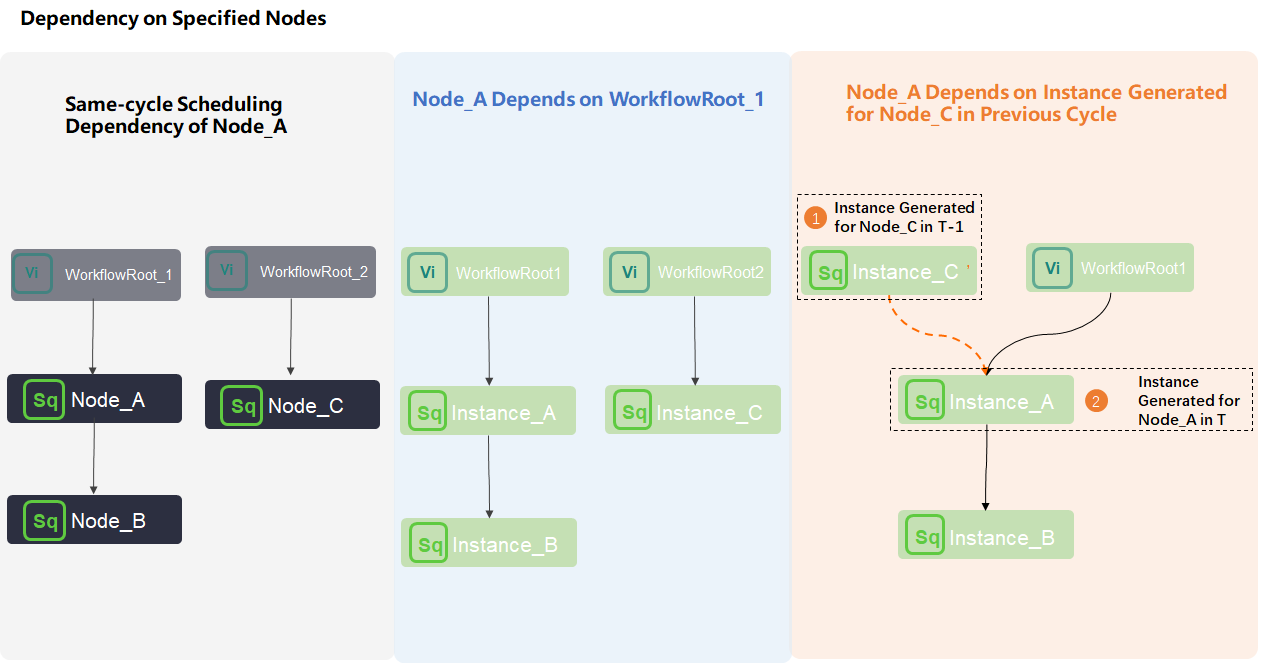After you set Depend On to Other Nodes for Node_A, Instance_A depends on Instance_C' and the instance generated for WorkflowRoot_1. In this case, Instance_A starts to run only after Instance_C' and the instance generated for WorkflowRoot_1 are successfully run.

Pass the dry-run attribute of an ancestor node

• Scenario
If a branch node has two descendant nodes, one descendant node can normally run, and the other is dry-run. If you configure the instance generated for the dry-run descendant node in the current cycle to depend on the instance generated for the dry-run descendant node in the previous cycle, the dry-run attribute of the node is passed to the descendant nodes of the dry-run node. In this case, all the instances generated for the dry-run node and the descendant nodes of the dry-run node are dry-run. If you do not want the dry-run attribute to be passed, you can set Follow the upstream air running attribute to No for the zero load node in the Dependencies section of the Properties tab.
Note The dry-run attribute of nodes other than branch nodes is not passed. When you use a branch node that has two or more descendant nodes, the dry-run attribute of one of the descendant nodes can be passed if you configure the instance generated for the descendant node in the current cycle to depend on the instance generated for the descendant node in the previous cycle.
• Example
• Assign_Node is an assignment node. Branch_Node is a branch node. Shell_Node1 and Shell_Node2 are the descendant nodes of Branch_Node. All these nodes are scheduled by day.
• Shell_Node1 is dry-run, and Shell_Node2 normally runs.
• The following configuration is performed for Shell_Node1: The instance generated in the current cycle depends on the instance generated in the previous cycle.
• Shell_Node1 generates an auto triggered node instance Shell_Node1' in the current cycle (T).
• Shell_Node1 generates an auto triggered node instance Shell_Node1 in the previous cycle (T-1).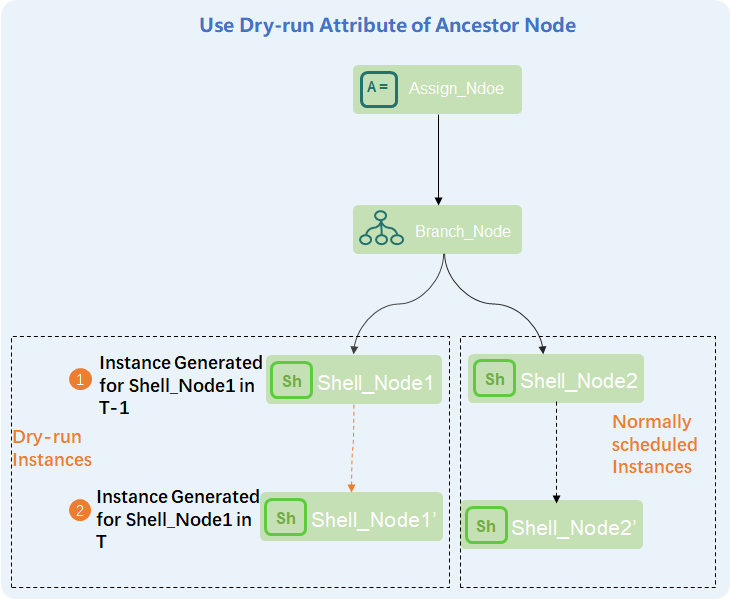Shell_Node1' depends on Shell_Node1. The dry-run attribute of Shell_Node1 is passed. Therefore, all the instances generated for Shell_Node1 and descendant nodes of Shell_Node1 are dry-run. You can set Follow the upstream air running attribute to No for Shell_Node1. This way, all the instances generated for Shell_Node1 and descendant nodes of Shell_Node1 can normally run.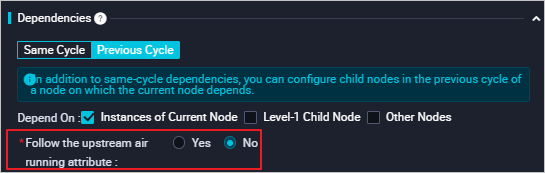Examples

For more information about typical scenarios of the dependency on instances in the previous cycle, see Scenario 2: Configure scheduling dependencies for a node that depends on last-cycle instances.